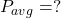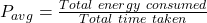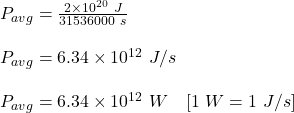Question

The total annual U.S. energy consumption is about 2×1020 joules. Part A What is the average power requirement for the United States, in watts? (Hint: 1 watt = 1 joule/s) Paverage = W

1.RuslanHeatt

6.34 × 10¹² W

Explanation:

Given:

Annual consumption of energy in U.S. (E) = 2 × 10²⁰ J

Average power requirement is,Now, we are given annual energy consumption. So, this means that, the energy consumption in a year is given.

Now, let us calculate the number of seconds in a year.

We know that,

1 year = 365 days

1 day = 24 hours

1 hour = 60 min

1 min = 60 s

Therefore, 1 year = 365 × 24 × 60 × 60 = 31536000 s

Now, the average power requirement in a year is given by the formula:Plug in the values given and solve for average power. This gives,Therefore, the average power requirement for the United States, in watts is 6.34 × 10¹² W